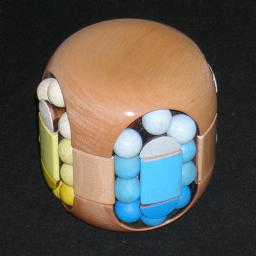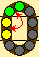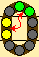# Rainbow PuzzleThis wooden puzzle by Clever Toys consists of a cylinder split into three layers. The top and bottom layers are connected by a central axle, around which the middle layer can rotate. The top and bottom layer cannot rotate with respect to each other. On the side of this cylinder there are four looped tracks containing balls. These tracks are vertical loops split across the three layers. The top and bottom layers contain curved sections of track, all holding three balls, while the middle layer contains vertical sections of track, (two from each loop) each containing two balls. The loop of 10 balls in a track can be moved around in unison. The neat thing is that the middle layer's eight track sections are equidistantly spaced, so that there are eight ways for the middle layer to be aligned, not just four as you would expect with the four loops.

In its solved state, the surface within each loop has a distinct colour (blue, red, green, yellow), with the top layer being a lighter shade of the relevant colour, and the bottom layer a darker shade. The balls in the loop of course have to match this in colour and shade.

If your browser supports JavaScript, then you can play the Rainbow Puzzle by clicking the link below:

## The number of positions:

There are 40 balls, 8 sets of three and 4 sets of four identically coloured ones. This gives 40! / (3!8·4!4) ball arrangements. This has to be multiplied by 8 due to the position of the middle layer, which gives a total of 8·40! / (3!8·4!4) = 11,713,322, 080,860,915,346,154, 459,136,000,000,000 = 1.17·1037 positions.

## Solution:

Phase 1: Solving the top and bottom of three loops.
I will assume that you are holding the puzzle so that the top layer has the light colour shades, and the bottom layer the dark shades.
In this phase, the light and dark balls of three of the colours will be solved. This is done in pairs, placing a light and a dark ball together at directly opposite positions of a loop. Note that any light or dark ball that is not part of such an opposing pair is considered unsolved, even if it happens to be in the correct section of track.

1. Choose a colour that you wish to solve.
2. Find an unsolved dark ball of your chosen colour.
3. Bring the dark ball to one of the loops that doesn't yet contain any solved balls. As you bring the dark ball to this spare loop, make sure you do not move any balls you previously solved out of their sections of track.
4. Rotate that spare loop so that the dark ball is in the top or bottom section of track.
5. Find a light ball of your chosen colour.
6. Bring the light ball to the spare loop, again not overly disturbing any balls you may have solved previously.
7. Move the middle layer to the right, so that the light ball is now no longer in the spare loop but in the vertical section directly to the right of it.
8. Rotate the spare loop so that the dark ball lies in the middle layer on the right hand side, but not at the same height as the light ball.
9. Move the middle layer left, so that both the light and the dark ball are in the spare loop. Note that they now lie opposite each other in the loop.
10. Put the pair of balls in place as follows:
1. If necessary, rotate the spare loop so that the light ball is in the middle layer on the right hand side, at the top of the vertical section, so that the dark ball is in the middle layer at the bottom of the left vertical section.
2. If necessary, rotate the loop you are solving so that its previously solved light balls are on the right hand end of the top section of track (the dark solved balls at the left hand end of the bottom section of track).
3. Move the middle layer to bring the pair of balls to the correct loop. The two balls should now be adjacent to any pairs that you solved previously.
4. Rotate the loop anti-clockwise one step, putting the new pair in the top/bottom sections of track.
11. Repeat steps b-j twice more so that all the light/dark balls of your colour are solved.
12. Repeat steps a-k twice more so the light/dark balls of three of colours are solved.

Phase 2: Solving the middle layer of three loops.
From now on the three partially solved loops will never be rotated. You will only rotate the remaining loop and move the middle layer.
In this phase, the middle balls of three of the colours will be solved. This is done by solving the six vertical sections of track one by one.

1. Hold the puzzle so that the only completely unsolved loop is at the front, and the middle layer is such that its coloured centres are in their correct positions.
2. Make note of the colour of the loop on the left. We will first solve the vertical section to the right of the centre with that colour.
3. Find an unsolved ball of the colour.
4. If the ball isn't already in the front ring, then move the middle layer to bring it to the right hand side of the front loop.
5. Turn the front loop so as to place the ball at the right end of the top section of track.
6. Turn the middle layer so that the centre of the same colour as the ball lies at the front.
7. Rotate the front loop clockwise one step, putting the ball into position at the top right of the centre.
8. Rotate the middle layer so that its coloured centres are in the right places again.
9. Repeat steps c-h once more, completing the first vertical section of that colour. Next, the second section of that colour is solved.
10. Find an unsolved ball of the colour.
11. If the ball isn't already in the front ring, then move the middle layer to bring it to the right hand side of the front loop.
12. Turn the front loop so as to place the ball at the right end of the top section of track.
13. Turn the middle layer so that the centre of the same colour as the ball lies just to the right of the front loop.
14. Rotate the front loop clockwise one step, putting the ball into position at the top left of the coloured centre.
15. Rotate the middle layer so that its coloured centres are in the right places again.
16. Repeat steps j-o once more, completing the second vertical section of that colour.
17. Do steps c-p again, this time for the colour of the rear loop (the one opposite the front unsolved loop).
18. Do steps c-p once more, this time for the colour of the right loop. This leaves only the front loop unsolved.

Phase 3: Solve the dark balls of the last loop.
For the last two phases, two closely related move sequences will be used.
 Move Description Short form Effect 1. 1. Shift middle layer to the left one step. 2. Rotate the front loop two balls anti-clockwise. 3. Shift middle layer to the left one step. 4. Rotate the front loop two balls clockwise. 5. Shift middle layer to the right one step. 6. Rotate the front loop two balls anti-clockwise. 7. Shift middle layer to the right one step. 8. Rotate the front loop two balls clockwise. L AA L CC R AA R CC Swaps the left vertical pair with the pair just above it.2. 1. Shift middle layer to the left one step. 2. Rotate the front loop three balls anti-clockwise. 3. Shift middle layer to the left one step. 4. Rotate the front loop three balls clockwise. 5. Shift middle layer to the right one step. 6. Rotate the front loop three balls anti-clockwise. 7. Shift middle layer to the right one step. 8. Rotate the front loop three balls clockwise. L AAA L CCC R AAA R CCC Swaps the left vertical pair with the second and third balls above it.1. If there is a pair of adjacent dark balls, then go to step d.
2. If there are two dark balls with two other balls between them, then rotate the loop so that the dark balls lie at the left of the top and bottom sections, and do move sequence 1 to bring them together. Continue with step d.
3. If there are two dark balls with three other balls between them (the middle one may be dark too), then rotate the loop so that one dark ball lies at the left of the bottom section and the other in the middle of the top section. Then do move sequence 2 to bring them together.
4. You now have an adjacent dark ball pair, and a third loose dark ball.
5. If there are two balls between the dark pair and the third dark ball, then rotate the loop so that the dark balls lie at the left of the top and bottom sections, and do move sequence 1 to bring them together. Note that this is the same as step a. Continue with step h.
6. If there are three balls between the dark pair and the third dark ball, then rotate the loop so that one dark ball lies at the left of the bottom section and another in the middle of the top section. Then do move sequence 2 to bring them together. Note that this is the same as step b. Continue with step h.
7. If there is one ball between the dark pair and the third dark ball, then rotate the loop so that one dark ball lies in the middle of the top section and another at the bottom of the left vertical section, and do move sequence 1 to bring them together.
8. Rotate the loop to put the three dark balls in their correct place, the bottom section of the loop.

Phase 4: Solve the light balls of the last loop.
We will again only use those two move sequences. As things are a little complicated to explain, I will number the (positions of the) remaining balls 1-7 clockwise, with 1 being at the bottom of the left vertical section, and 7 at the bottom of the right vertical section. With this numbering scheme, the first move sequence swaps 1&2 with 3&4, and the second swaps 1&2 with 4&5. Note however that by temporarily rotating the loop first, we can swap any adjacent pairs with the first sequence, and any two pairs that are 1 ball apart using the second sequence.

1. If there is a light ball at 1 then:
if position 4 does not have a light ball, do the second sequence,
otherwise, do the first sequence.
Repeat if necessary until the ball at 1 is not light coloured.
2. If there is a light ball at 7 then:
if position 4 does not have a light ball, use the second sequence to swap 3&4 with 6&7,
otherwise, do the first sequence to swap 4&5 with 6&7.
Repeat if necessary until the ball at 7 is not light coloured.
3. If there are light balls at both 2 and 6, then use the second sequence to swap 2&3 with 5&6.
4. If the light balls are at 2, 3, and 4, then use the first sequence to swap 2&3 with 4&5.
5. If the light balls are at 4, 5, and 6, then use the first sequence to swap 3&4 with 5&6.
6. If the light balls are at 2, 4, and 5, then use the second sequence to swap 1&2 with 4&5, and then the first sequence to swap 1&2 with 3&4.
7. If the light balls are at 3, 4, and 6, then use the second sequence to swap 3&4 with 6&7, and then the first sequence to swap 4&5 with 6&7.
8. If the light balls are at 2, 3, and 5, then use the first sequence to swap 2&3 with 4&5.
9. If the light balls are at 3, 5, and 6, then use the first sequence to swap 3&4 with 5&6.